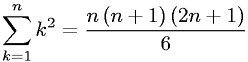Equations > Calculus > Sequences and Series > Arithmetic Series - Sequential Squared Integers

### Arithmetic Series - Sequential Squared IntegersLatex Code:

MathML Code:

 $\sum _{k=1}^{n}{k}^{2}=\fracn\left(n+1\right)\left(2n+1\right)6$

MathType 5.0: